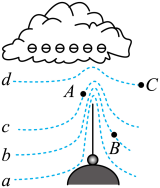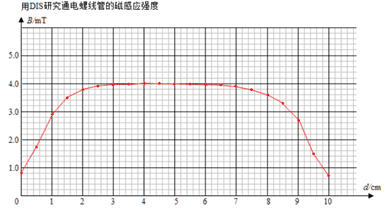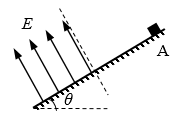## 长宁区2022学年第一学期期末

•  2022/12/18
•  200 次
•  285 K

1．下列各组能源中，均属于一次能源的是

（A）水能、天然气、太阳能                              （B）风能、煤气、太阳能

（C）水能、电能、天然气                                   （D）风能、电能、煤气

【答案】

A

2．在“用油膜法估测分子的大小”实验中，估测的物理量是

（A）水分子的直径                                               （B）油酸分子的直径

（C）水分子的体积                                                （D）油酸分子的体积

【答案】

B

3．右图为甲、乙两物体沿同一直线运动的位移 s 随时间 t 变化的图像。下列说法中正确的是

（A）两物体的运动方向始终相同

（B）乙物体做匀加速直线运动

（C）t1 时刻两物体速度相等

（D）t1 ~ t2 时间内两物体平均速度相等

【答案】

D20

4．声波能绕过某一建筑物传播而光波却不能绕过该建筑物，这是因为

（A）声波穿透力比光波强                                   （B）声波是纵波，光波是横波

（C）声波波长较长，光波波长很短                  （D）声波波速较小，光波波速很大

【答案】

C

5．用电动势为 E、内阻为 r 的电源对外电路供电，则

（A）电源短路时，路端电压为最大值

（B）外电路断开时，路端电压为零

（C）路端电压增大时，流过电源的电流一定减小

（D）路端电压增大时，外电路消耗的功率一定增大

【答案】

C

6．两个相同的单摆静止于平衡位置，使摆球分别以水平初速 v1v2v1 > v2）离开平衡位置，在竖直平面内做小角度摆动，它们的周期与振幅分别为 T1T2A1A2、，则

（A）T1 = T2A1 > A2                           （B）T1 < T2A1 = A2

（C）T1 = T2A1 < A2                           （D）T1 > T2A1 = A2

【答案】

A

7．雷雨天带有负电的乌云飘过一栋建筑物上空时，在避雷针周围形成电场。该电场的等势面 a、b、c、d 分布情况如图所示，在等势面上有 A、B、C 三点。下列说法中正确的是

（A）避雷针附近形成了匀强电场

（B）A、B、C 三点中，B 点场强最大

（C）A、B、C 三点中，C 点电势最高

（D）一带负电的雨点从乌云中下落，电场力做正功

【答案】

D

8．物体做自由落体运动，Ek 为动能，Ep 为势能，s 为下落的距离，v 为速度，t 为时间。以水平地面为零势能面，下列图像中，正确反映各物理量之间关系的是【答案】

A

【解析】

A．Ep = mgh = mgh0s），为斜率为负的倾斜直线。选项 A 正确；

B．由机械能守恒定律 E0 = Ek + EpEp = − Ek + E0，为斜率为负的倾斜直线。选项 B 错误；

C．Ek = $$\frac{1}{2}$$mv2 = $$\frac{1}{2}$$m(gt)2，为斜率为正的倾斜直线。选项 C 错误；

D．Ep = mgh = mgh0 − $$\frac{{{v^2}}}{{2g}}$$），为开口向下的抛物线。选项 D 错误；

9．如图所示，将长为 2L 的直导线折成边长相等、夹角为 60° 的“V”形，并置于与其所在平面垂直的、磁感应强度为 B 的匀强磁场中。若“V”形导线所受的安培力大小为 F，则该直导线中通过的电流大小为

（A）$$\frac{F}{{2BL}}$$             （B）$$\frac{{\sqrt 3 F}}{{2BL}}$$            （C）$$\frac{F}{{BL}}$$              （D）$$\frac{{\sqrt 3 F}}{{BL}}$$

【答案】

C

10．某人骑自行车沿平直坡道向下滑行，其车把上挂有一只水壶，壶内装有半瓶水。若滑行过程中悬挂壶的细绳始终保持竖直，不计空气阻力，则下列说法正确的是

（A）自行车可能做匀加速运动

（B）壶内水面与坡面平行

（C）坡道对自行车的作用力垂直坡面向上

（D）水壶及水整体的重心一定在悬绳正下方

【答案】

D

11．如图所示，两端开口、内径均匀的玻璃弯管固定在竖直平面内，两段水银柱 A 和 C 将空气柱 B 封闭在左侧竖直段玻璃管，平衡时 A 段水银有一部分在水平管中，竖直部分高度为 h2，C 段水银两侧液面高度差为 h1。若保持温度不变，向右管缓缓注入少量水银，则再次平衡后

（A）空气柱 B 的长度减小               （B）左侧水银面高度差 h2 减小

（C）空气柱 B 的压强增大               （D）右侧水银面高度差 h1 增大

【答案】

B

【解析】

B 部分气体的压强可以表达为 p0 + ρgh2，因此 B 部分气体压强减小，体积（长度）变大。选项 A、C 错误；

12．一列波长大于 3 m 的横波沿着 x 轴正方向传播，处在 x1 = 1.5 m 和 x2 = 4.5 m 的两质点 A、B，当 B 点的位移为正的最大值时，A 点的位移恰为零，且向上运动。以该时刻为计时起点，t = 1.0 s 时，A 点处于波峰。则

（A）波长为 12 m                                         （B）波速可能为 5 m/s

（C）1 s 末 A、B 两点的位移相同          （D）2 s 末 A 点的运动速度小于 B 点的运动速度

【答案】

B

【解析】满足“当 B 点的位移为正的最大值时，A 点的位移恰为零，且向上运动”的波形如图所示。

A．由图可知，$$\frac{3}{4}$$ λ = 3 m，λ = 4 m。选项 A 错误；

B．由“t = 1.0 s 时，A 点处于波峰”可知 (n + $$\frac{1}{4}$$)T = 1，解得波速 v = $$\frac{\lambda }{T}$$ = 4n + 1，当 n = 1 时，v = 5 m/s。选项 B 正确；

C．1 s 末 A 位于波峰、B 位于平衡位置，两者位移不同。选项 C 错误；

D．2 s 末 A 位于平衡位置速度最大、B 位于波谷速度为零，A 点的运动速度大于 B 点的运动速度。选项 D 错误；

13．内能是物体内部具有的能量，它包括物体内所有分子的__________，它与物体的__________、状态都有关系。

【答案】

14．在“用 DIS 研究通电螺线管的磁感应强度”实验中，在螺线管通电前，要先对磁传感器进行____________。实验中得到如图所示的 B d 图线，若螺线管匝数为 100 匝，其内径（直径）为 4 × 10−2 m，根据图线估算螺线管中心的磁通量约为_________Wb。

【答案】

15．甲、乙两个物体在水平面内做半径之比为 8∶9 的匀速圆周运动。在相同时间内，它们通过的路程之比为 4∶3，运动方向改变的角度之比为 3∶2。则它们的周期之比为_______，向心加速度之比为_______。

【答案】

2∶3，2∶1

16．物体从静止开始沿光滑的固定斜面加速下滑，以 t 为时间间隔，在第一个 t 内物体获得动能为 ∆Ek，在第 3 个 t 内，物体动能的增量为______；在这 3t 时间内，重力对物体做功的平均功率为_______。

【答案】

5∆Ek，$$\frac{{3\Delta {E_k}}}{t}$$

17．如图，倾角为 θ 的斜面粗糙且绝缘，在虚平面下方区域有一垂直斜面向上的匀强电场。一质量为 m、电荷量为 q 的带负电的小物块（可视为质点），从斜面上 A 点以速度 v0 沿斜面匀速下滑，进入电场区域滑行距离 L 后停止，则匀强电场场强 E = ______。在电场中滑行 L 的过程中，带电小物块电势能的变化量 ∆Ep = _________。

【答案】

$$\frac{{mv_0^2}}{{2Lq\tan \theta }}$$，0

【解析】

（1）小物块在斜面上匀速运动时，有

mgsinθμmgcosθ = 0

（2）物块在电场中运动时，运动方向垂直于电场强度的方向，电场力不做功，因此电势能的变化量为零。

18．“用 DIS 描绘电场的等势线”的实验示意图如图所示。图中 A、B 是连接电源的两个电极，基准点 c 位于 A、B 连线的中点，f、d 连线和 A、B 连线垂直。

（1）（单选）安装实验装置时，正确的做法是

（A）平整的木板上依次铺放白纸、导电纸、复写纸

（B）导电纸有导电物质的一面向上

（C）电源电压为交流 4 ~ 6 V

（D）电极 A、B 必须与导电物质保持绝缘

（2）实验中如果忘记传感器调零，则描绘得到的等势线形状与原来的_________。（选填“相同”或“不同”）

（3）（单选）实验中得到的曲线实际上是

（A）一对等量同种电荷的静电场的等势线                      （B）稳恒电流场中的等势线

（C）一对等量异种电荷的静电场的等势线                      （D）变化电流场中的等势线

（4）若电压传感器的红、黑色探针分别接触图中的 d、f 两点时，示数小于零，则可以判断电极 A 接在电源的_____极上（选填“正”或“负”）；为使传感器示数为零，应保持红色探针与 d 点接触，将黑色探针_______（选填“向左”或“向右”）移动。

【答案】

（1）B

（2）不同

（3）B

（4）正，向右

19．如图所示，电阻 R3 = 4 Ω，电表均为理想表。开始时 R1R3 均有电流通过，电压表示数为 2 V，电流表示数为 0.75 A。若某个电阻发生断路，使电压表示数变为 3.2 V，电流表示数变为 0.8 A。

（1）分析判断发生断路的是哪个电阻。

（2）R1R2 的阻值各为多少？

（3）电源的电动势 E 和内阻 r 各为多少？

【答案】

（1）R2

（2）R1 = 4 Ω，R2 = 8 Ω

（3）E = 4 V，r = 1 Ω

【解析】（1）假如 R1 断路，则电流表示数为 0，不符合；假如 R3 断路，则电压表示数为 0，也不符合，所以 R2 断路。

（2）R2 断路时，电压表与 R3 串联，相 R3 当于导线，等效电路如图，电压表读数为 R1 的电压值，故

R1 = = Ω = 4 Ω

R2 发生断路前，通过 R3 的电流为：

I3 = = A = 0.25 A

（3）由全电路欧姆定律 E = U + Ir

E = 3 + (0.75 + 0.25)r

E = 3.2 + 0.8r

20．如图所示，倾角 θ = 30° 的光滑斜面上，放置了一装有挡光片的滑块 A，其质量 m = 0.2 kg。A 在恒力 F 作用下以一定初速度沿斜面向上做匀减速直线运动，速度减为零后又继续沿斜面下滑。当 A 滑到粗糙水平面 O 点时撤去 F，此时完全相同的滑块 B 以一定初速度沿水平面向右滑行。a、b、c、d、e 是五个光电门，测量滑块通过所在位置的速率。忽略滑块 A 过 O 点时的能量损失，以滑块 A 向上经过光电门 a 的瞬间为 t1 = 0 时刻，每个光电门在各个时刻测得的速率记录在下表（其中 v3 数据不详），g 取 10 m/s2光电门编号       速度（m/s） 时刻（s）

a

b

c

d

e

t1 = 0.0

v1 = 2

t2 = 0.8

v2 = 2

t3 = 1.0

v3 =

t4 = 1.2

v4 = 1

v5 = 2

t5 = 2.4

v6 = 0.5

（1）计算滑块 A 在斜面上运动时的加速度。

（2）t3 = 1.0 时，v3 的数值是多少？

（3）以光电门 b 的位置为零势能面，计算滑块 A 通过光电门 a 的机械能，分析说明滑块 A 在斜面上运动时机械能是否守恒。

（4）光电门 e、c 间距 L = 2 m，若 A、B 两滑块在 t5 时刻前还未相遇，通过分析判断光电门 d 测得的是哪个滑块的速度。

【答案】

（1）a = 5 m/s2

（2）v3 = 3 m/s

（3）Ea = 0.9 J

（4）d 传感器测到的是 B 滑块速率

【解析】

（1）滑块 A 在斜面上运动时所受合力不变，做匀减速直线运动，加速度沿斜面向下。从表格中前两组数据可知，滑块 A 从斜面最高点滑至光电门 a 的时间间隔为 Δt = 0.4 s

a = $$\frac{{\Delta v}}{{\Delta t}}$$ = $$\frac{{{v_2} - 0}}{{\frac{{{t_2} - {t_1}}}{2}}}$$ = $$\frac{2}{0.4}$$ = 5 m/s2          方向沿斜面向下

（2）v3 是滑块A滑至光电门 b 的速度，v3 = v2 + at = 2 + 5×(1.0 – 0.8) m/s = 3 m/s

（3）sab = $$\frac{{v_3^2 - v_2^2}}{{2a}}$$ = 0.5 m     斜面倾角 θ = 30°  得 hab = 0.25 m

（4）A、B 两相同滑块在水平面上受力相同，由牛顿第二定律，加速度大小相同。

a = $$\frac{{{v_6} - {v_4}}}{{{t_5} - {t_4}}}$$ = − $$\frac{5}{12}$$ m/s2

B 滑块位移为 sB = $$\frac{{{v_4} + {v_6}}}{2}$$ (t5t4) = 2.1 m

a = $$\frac{{{v_6} - {v_5}}}{{{t_5} - {t_4}}}$$ = − 1.25 m/s2

B 滑块位移为 sB = $$\frac{{v_6^2 - v_5^2}}{{2a}}$$ = 1.5 m

2006 - 2023，推荐分辨率1024*768以上，推荐浏览器Chrome、Edge等现代浏览器，截止2021年12月5日的访问次数：1872万9823 站长邮箱